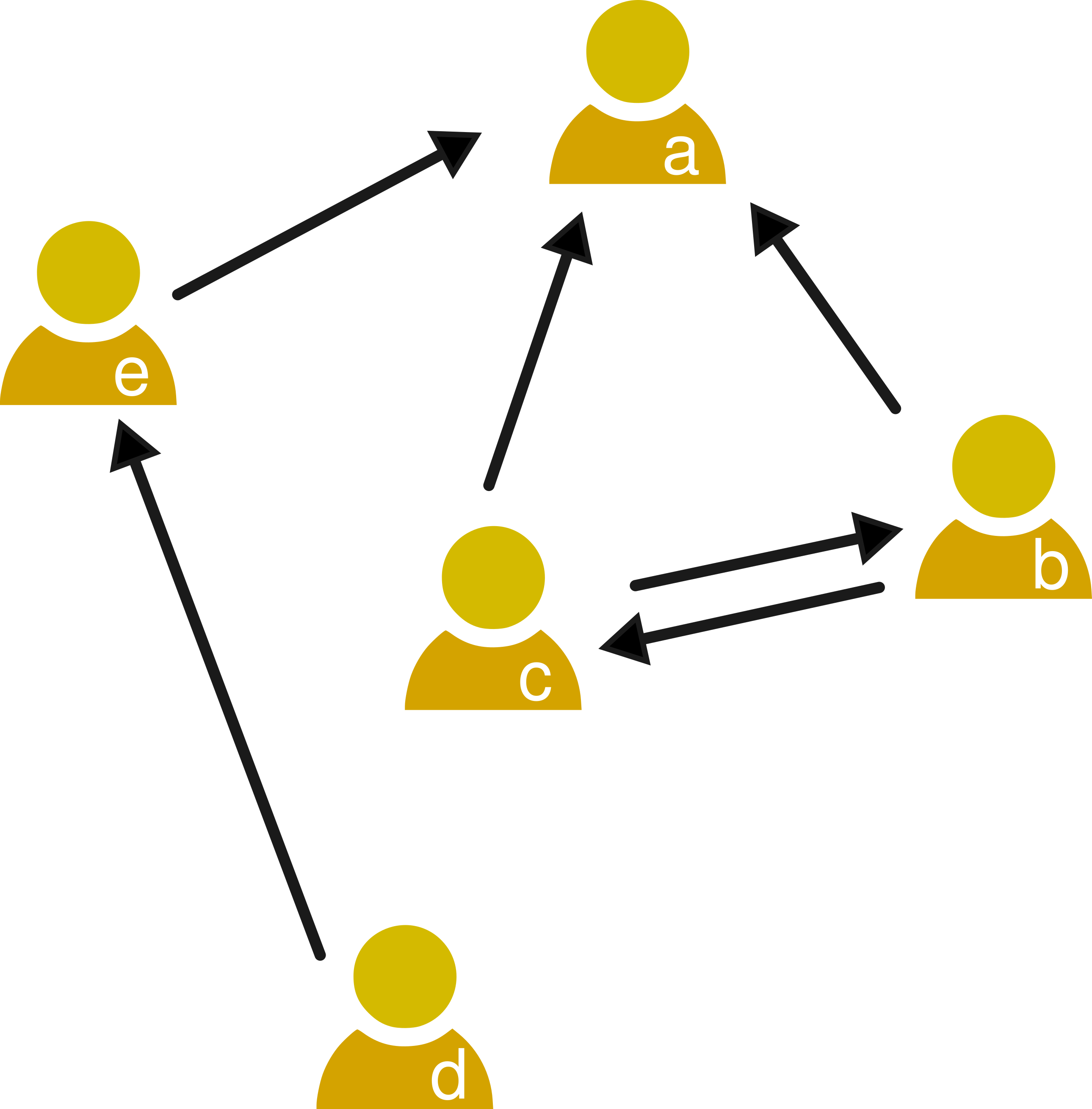### The concept of centrality

A common question when analyzing organizations or societies is how important an individual is. Social network analysis can be used to measure the importance of a person as a function of the social structure of a community or organization. In social networks, centrality measures this kind of structural importance of the node of a person. There are various centrality measures that stem from different kinds of structural importance. In this topic you will learn about three centrality measures:

1. Degree centrality
2. Betweenness centrality
3. Closeness centrality

In the social resilience topic you can learn about a fourth centrality topic, coreness centrality.

### Degree centralityThe importance of a node might grow with number of other nodes you can reach from it. In this case, degree centrality can be used to measure importance. As we saw in the introduction to social networks, a node’s degree measures the number of links connected to it. In undirected networks, there is only one measure of degree \(d(i)\), which is exactly the number of edges connected to the node \(i\). This can be a measure of importance for example in a friendship network like we saw for the friendship paradox, where importance is the number of friends of a person.

In directed networks there are two kinds of degree: in-degree \(d_{in}(i)\) that is the number of edges ending in \(i\), i.e. \((j,i)\) and out-degree \(d_{out}(i)\) that is the number of edges leaving from \(i\), i.e. \((i,j)\). In the network example of the right, \(d_{in}(c) = 1\) and \(d_{out}(c) = 2\). If importance on Twitter is the number of followers of an account, in-degree centrality is a way to measure it. An example of out-degree centrality as a measure of importance is an information forwarding network in an organization, where the person that forwards information to the most people would be the most important.

The weight of a link can play a role in the calculation of importance, for example when the strength of social relationships is measured in a social network. In weighted networks you can have the measures of weighted in-degree centrality and weighted out-degree centrality, which are the sum of the incoming and outgoing weights of a node respectively.